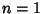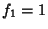## TreeA tree is a mathematical structure which can be viewed as either a Graph or as a Data Structure. The two views are equivalent, since a tree Data Structure contains not only a set of elements, but also connections between elements, giving a tree graph.

A tree graph is a set of straight line segments connected at their ends containing no closed loops (cycles). A tree withnodes hasEdges. The points of connection are known as Forks and the segments as Branches. Final segments and the nodes at their ends are called Leaves. A tree with two Branches at each Fork and with one or two Leaves at the end of each branch is called a Binary Tree.

When a special node is designated to turn a tree into a Rooted Tree, it is called the Root (or sometimes Eve.'') In such a tree, each of the nodes which is one Edge further away from a given node is called a Child, and nodes connected to the same node which are the same distance from the Root are called Siblings.

Note that two Branches placed end-to-end are equivalent to a single Branch which means, for example, that there is only one tree of order 3. The numberof nonisomorphic trees of order, 2, ... (where trees of orders 1, 2, ..., 6 are illustrated above), are 1, 1, 1, 2, 3, 6, 11, 23, 47, 106, 235, ... (Sloane's A000055).

Otter showed that(1)

(Otter 1948, Harary and Palmer 1973, Knuth 1969), where the constantsandare sometimes called Otter's Tree Enumeration Constants. Write the Generating Function for Rooted Trees as(2)

where the Coefficients are(3)

withand. Then(4)

is the unique Positive Root of(5)

and(6)

See also B-Tree, Binary Tree, Caterpillar Graph, Cayley Tree, Child, Dijkstra Tree, Eve, Forest, Kruskal's Algorithm, Kruskal's Tree Theorem, Leaf (Tree), Orchard-Planting Problem, Ordered Tree, Path Graph, Planted Planar Tree, Pólya Enumeration Theorem, Quadtree, Red-Black Tree, Root (Tree), Rooted Tree, Sibling, Star Graph, Stern-Brocot Tree, Weakly Binary Tree, Weighted Tree

References

Finch, S. Favorite Mathematical Constants.'' http://www.mathsoft.com/asolve/constant/otter/otter.html

Chauvin, B.; Cohen, S.; and Rouault, A. (Eds.). Trees: Workshop in Versailles, June 14-16, 1995. Basel, Switzerland: Birkhäuser, 1996.

Gardner, M. Trees.'' Ch. 17 in Mathematical Magic Show: More Puzzles, Games, Diversions, Illusions and Other Mathematical Sleight-of-Mind from Scientific American. New York: Vintage, pp. 240-250, 1978.

Harary, F. and Manvel, B. Trees.'' Scripta Math. 28, 327-333, 1970.

Harary, F. and Palmer, E. M. Graphical Enumeration. New York: Academic Press, 1973.

Knuth, D. E. The Art of Computer Programming, Vol. 1: Fundamental Algorithms, 2nd ed. Reading, MA: Addison-Wesley, 1973.

Otter, R. The Number of Trees.'' Ann. Math. 49, 583-599, 1948.

Sloane, N. J. A. Sequence A000055/M0791 in An On-Line Version of the Encyclopedia of Integer Sequences.'' http://www.research.att.com/~njas/sequences/eisonline.html and extended entry in Sloane, N. J. A. and Plouffe, S. The Encyclopedia of Integer Sequences. San Diego: Academic Press, 1995.